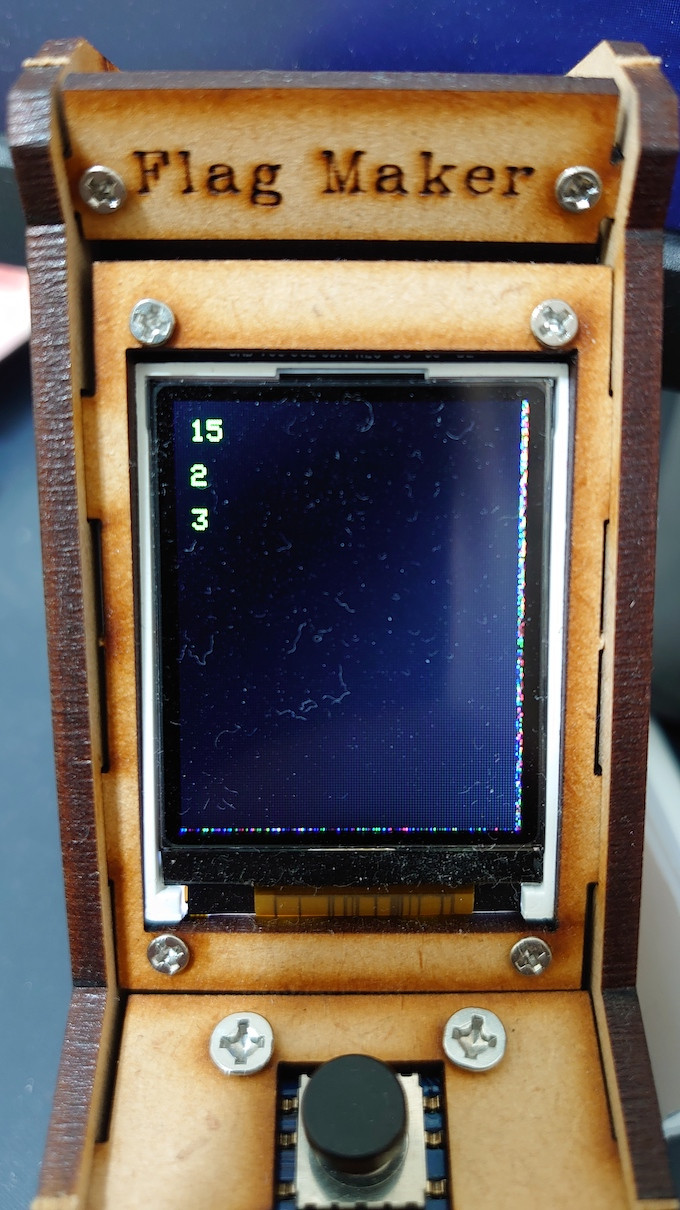#DAY 20
0
IoT

## TFT_eSPI 套件

TFT_eSPI 是我自己嘗試過比較不會出問題的套件，同時也支援好幾種市面上能取得的螢幕，要使用這個套件可以透過 PlatformIO 的 `lib_deps` 選項追加。

``````; platformio.ini

; ...

[env:d1]
platform = espressif8266
board = d1
framework = arduino
custom_ruby_script = app.rb
build_flags = -D ESP8266
test_ignore = test_*
lib_deps =
TFT_eSPI
``````

## 螢幕設定

``````; platformio.ini

; ...

build_flags =
-DESP8266
-Os
-DST7735_DRIVER=1
-DTFT_WIDTH=128
-DTFT_HEIGHT=160
# PIN configure
-DTFT_MISO=12
-DTFT_MOSI=13
-DTFT_SCLK=25
-DTFT_CS=15
-DTFT_DC=5
-DTFT_RST=-1
-DSPI_FREQUENCY=40000000
``````

## 繪製文字

``````// src/main.c

#if defined(ESP8266)
#include <Arduino.h>
#include <TFT_eSPI.h>
#include <SPI.h>
#endif
``````

``````// src/main.c

// ...

#if defined(ESP8266)
TFT_eSPI tft = TFT_eSPI();
#endif

void mrb_puts(mrb_state* mrb) {
int argc = mrb_get_argc(mrb);
mrb_value* argv = mrb_get_argv(mrb);

#if defined(ESP8266)
char num;
tft.fillScreen(TFT_BLACK);
#endif

for(int i = 0; i < argc; i++) {
printf("%d\n", mrb_fixnum(argv[i]));
#if defined(ESP8266)
sprintf(num, "%d", mrb_fixnum(argv[i]));
tft.drawString(num, 8, i * 16 + 8, 1);
#endif
}
}
``````

``````void setup() {
Serial.begin(9600);

tft.init();
tft.setTextSize(1);
tft.setTextColor(TFT_GREEN, TFT_BLACK);

run_vm();
}
``````